Related Articles

# How to Hide Axis Text Ticks or Tick Labels in Matplotlib?

• Last Updated : 17 Dec, 2020

Prerequisites: Matplotlib

The Matplotlib library by default shows the axis ticks and tick labels. Sometimes it is necessary to hide these axis ticks and tick labels. This article discusses some methods by which this can be done.

### Functions used:

• xticks(ticks=None, labels=None, **kwargs)– used to get and set the current tick locations and labels of the x-axis.
• yticks(ticks=None, labels=None, **kwargs)- used to get and set the current tick locations and labels of the y-axis.
• set_visible(boolean)- sets visibility

### Hiding tick labels

Method 1:

The functions xticks() and yticks() are used to denote positions using which a data point is supposed to be displayed. They take a list as argument. Thus, axis text ticks or tick labels can be disabled by setting the xticks and yticks to an empty list as shown below:

```plt.xticks([])
plt.yticks([])```

Example 1:

## Python3

 `import` `matplotlib.pyplot as plt`` ` `x1 ``=` `[``5``, ``8``, ``10``]``y1 ``=` `[``12``, ``16``, ``8``]``x2 ``=` `[``6``, ``9``, ``12``]``y2 ``=` `[``14``, ``10``, ``8``]`` ` `plt.plot(x1, y1, ``'g'``, linewidth``=``7``)``plt.plot(x2, y2, ``'b'``, linewidth``=``7``)`` ` `plt.title(``'Disabling xticks and yticks'``, fontsize``=``20``)`` ` `plt.xlabel(``'X axis'``, fontsize``=``15``)``plt.ylabel(``'Y axis'``, fontsize``=``15``)`` ` `# disabling xticks by Setting xticks to an empty list``plt.xticks([])  `` ` `# disabling yticks by setting yticks to an empty list``plt.yticks([])  `` ` `plt.show()`

Output: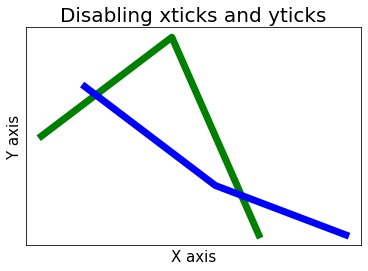Method 2:

By default, in matplotlib library, plots are plotted on a white background. Therefore, setting the color of tick labels as white can make the axis tick labels as hidden. For this only color attribute needs to passed with w (represents white) as a value to xticks() and yticks() function. Implementation is given below:

Example 2:

## Python3

 `import` `matplotlib.pyplot as plt`` ` `plt.plot([``5``, ``10``, ``20``], [``20``, ``10``, ``50``], color``=``'g'``)`` ` `plt.xlabel(``"X Label"``)``plt.ylabel(``"Y Label"``)`` ` `# xticks color white``plt.xticks(color``=``'w'``)`` ` `# yticks color white``plt.yticks(color``=``'w'``)`` ` `plt.show()`

Output: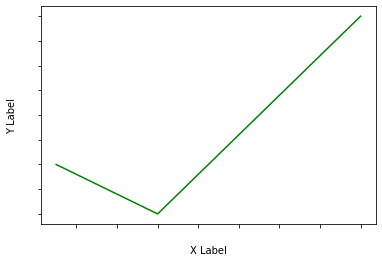Method 3:

Null Locator is a type of tick locator which makes the axis ticks and tick labels disappear. Simply passing NullLocator() function will be enough.

Example3:

## Python3

 `import` `numpy as np``import` `matplotlib.ticker as ticker`` ` `ax ``=` `plt.axes()`` ` `x ``=` `np.random.rand(``100``)``ax.plot(x, color``=``'g'``)`` ` `ax.xaxis.set_major_locator(ticker.NullLocator())``ax.yaxis.set_major_locator(ticker.NullLocator())`

Output: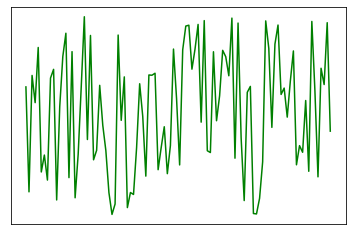Method 4:

Observe the syntax of xticks() and yticks() carefully, if even just a space is passed to them along with the data the output will be our desired result.

Example 4:

## Python3

 `import` `matplotlib.pyplot as plt`` ` `x ``=` `[``5``, ``8``, ``15``, ``20``, ``30``]``y ``=` `[``15``, ``10``, ``8``, ``20``, ``15``]``plt.plot(x, y, color``=``'g'``, linewidth``=``5``)`` ` `# x-label as blank``plt.xticks(x, ``" "``)`` ` `# y-label as blank``plt.yticks(y, ``" "``)`` ` `plt.show()`

Output: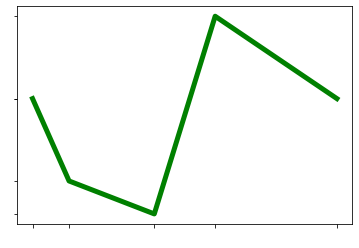Method 5:

This method is just a modification of method 4. Instead of passing an empty string, assign label as space in the functions itself.

Example 5:

## Python3

 `import` `matplotlib.pyplot as plt`` ` `x ``=` `[``5``, ``8``, ``15``, ``20``, ``30``]``y ``=` `[``15``, ``10``, ``8``, ``20``, ``15``]``plt.plot(x, y, color``=``'g'``, linewidth``=``5``)`` ` `plt.xticks(x, labels``=``" "``)``plt.yticks(y, labels``=``" "``)`` ` `plt.show()`

Output: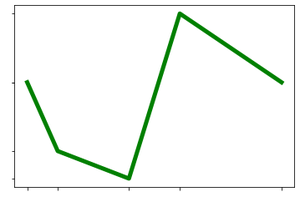Method 6:

Using set_visibile() we can also set visibility of tick labels as False, that will not make them appear in our plot. This method hides labels as well as ticks, so if some requirement needs ticks to be displayed this isn’t the option to for, multiple methods shown above would stand ideal though.

Example 6:

## Python3

 `import` `matplotlib.pyplot as plt`` ` `x ``=` `[``5``, ``8``, ``15``, ``20``, ``30``]``y ``=` `[``15``, ``10``, ``8``, ``20``, ``15``]``plt.plot(x, y, color``=``'g'``, linewidth``=``5``)`` ` `# getting current axes``a ``=` `plt.gca()`` ` `# set visibility of x-axis as False``xax ``=` `a.axes.get_xaxis()``xax ``=` `xax.set_visible(``False``)`` ` `# set visibility of y-axis as False``yax ``=` `a.axes.get_yaxis()``yax ``=` `yax.set_visible(``False``)`` ` `plt.show()`

Output: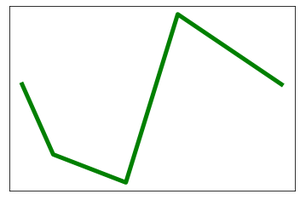Attention geek! Strengthen your foundations with the Python Programming Foundation Course and learn the basics.

To begin with, your interview preparations Enhance your Data Structures concepts with the Python DS Course. And to begin with your Machine Learning Journey, join the Machine Learning – Basic Level Course

My Personal Notes arrow_drop_up